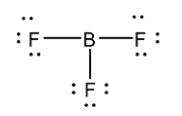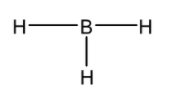QuestionAnswers

# Which of the following is not electron deficient?(a) ${ NH }_{ 3 }$(b) ${ BF }_{ 3 }$(c) ${ AlCl }_{ 3 }$(d) ${ BH }_{ 3 }$

Hint: Here we need to determine the octet and/or duplet (if applicable) of each atom in the molecule. If the octet and/or duplet (if applicable) of each atom in the molecule is complete then the molecule is not electron deficient.

In order to determine whether the molecule is electron deficient or not, we should determine whether the octet and/or duplet (if applicable) of each atom in the molecule is complete or not. If the octet and/or duplet (if applicable) of each atom in the molecule is complete then the molecule is not electron deficient. In order to determine whether the octet of a molecule is complete, we have to determine its chemical structure. To draw the chemical structure of the molecule, we have to take into account the VSEPR theory and the hybridisation theory.
Hybridization is achieved through linear combination of atomic orbitals and the new orbitals are called hybrid orbitals. In order to determine the hybridization of an atom in a molecule we should follow the following steps:
-First you have to determine the Lewis structure of the molecule.
-Now assign the regions of electron density around the atom using VSEPR theory for predicting the shape of the molecule (single bonds, multiple bonds, radicals, and lone pairs each will count as one region).
-Now we can determine the set of hybridized orbitals corresponding to the geometry determined suing VSEPR theory.
Let us determine the hybridisation in ${ NH }_{ 3 }$. Its Lewis structure is given below:From the above structure, it is clear that the regions of electron density around the central atom are four. Therefore the geometry of the molecule is tetrahedral according to VSEPR theory. For tetrahedral geometry the central atom must undergo ${ sp }^{ 3}$ hybridization. The 2s and three 2p orbitals of nitrogen combine together in order to give four ${ sp }^{ 3}$ hybrid orbitals. Our of these four hybrid orbitals, one contains a lone pair, therefore the shape of the molecule will be Trigonal pyramidal.
In the structure, the octet of nitrogen is complete and the duplet of hydrogen is also complete. Therefore this molecule is not electron deficient.
Now let us consider ${ BF }_{ 3 }$ molecule. Its Lewis structure is given belowFrom the above structure, it is clear that the regions of electron density around the central atom are three. Therefore the geometry of the molecule is Trigonal planar according to VSEPR theory. For Trigonal planar geometry the central atom must undergo ${ sp }^{ 2}$ hybridization.
In the structure, the octet of Boron is incomplete while the octet of all the Fluorine atoms is complete. Therefore this molecule is electron deficient.
Now let us look at ${ AlCl }_{ 3 }$ molecule. Its Lewis structure is given below:From the above structure, it is clear that the regions of electron density around the central atom are three. Therefore the geometry of the molecule is Trigonal planar according to VSEPR theory. For Trigonal planar geometry the central atom must undergo ${ sp }^{ 2}$ hybridization.
In the structure, the octet of Aluminium is incomplete while the octet of all the Chlorine atoms is complete. Therefore this molecule is electron deficient.
Now let us look into ${ BH }_{ 3 }$ molecule. Its Lewis structure is given below:From the above structure, it is clear that the regions of electron density around the central atom are three. Therefore the geometry of the molecule is Trigonal planar according to VSEPR theory. For Trigonal planar geometry the central atom must undergo ${ sp }^{ 2}$ hybridization.
In the structure, the octet of Boron is incomplete while the duplet of all the hydrogen atoms is complete. Therefore this molecule is electron deficient.

Hence the correct answer is (a) ${ NH }_{ 3 }$.

Note:
For Hydrogen atom, we need to consider the completion of its duplet rather than its octet since Hydrogen atom only has 1s orbital which can accommodate only two electrons. Also, ${ AlCl }_{ 3 }$ is not an ionic compound but rather a covalent compound because of the very high covalent character in the compound according to Fajan’s rules.